">

# Match the values of r with the accompanying scatterplots 0.723,-0.353,0.353,0.998 and -0.998 scatterplots 1,2,3,4,5Question
ScatterplotsMatch the values of r with the accompanying scatterplots 0.723,-0.353,0.353,0.998 and -0.998
scatterplots 1,2,3,4,5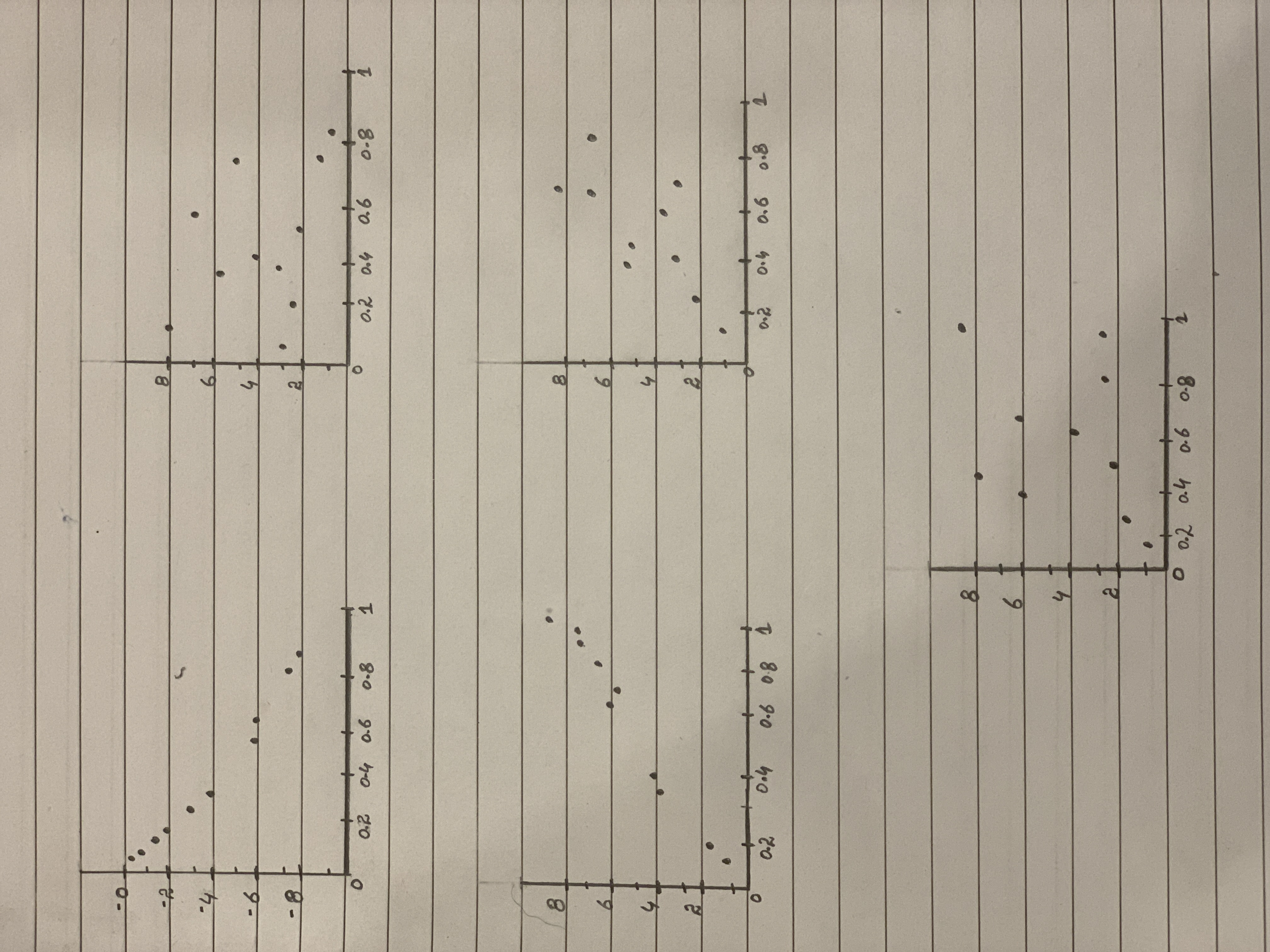2021-03-06
Correlation coefficient:
The value of correlation coefficient (r) ranges from –1 to 1.
The value of r closer to –1 or 1 indicates that there is strong correlation between two quantitative variables, whereas, the value of r closer to 0 indicates that there is weak or no correlation between variables.
The positive value of correlation indicates that both the variables (say x and y) are in same direction, whereas, the negative value of correlation indicates that both the variables are in opposite direction.
Match the following:
In scatter plot 1, the variables x and y are in opposite direction and it is approximately linear. Therefore, it has highest negative correlation. Among the given correlation values, it is clear that the r value for the scatter plot 1 is –0.998.
Similarly, by comparing the scatter plots with the r values, the scatterplots are matched with their respective r values as shown below:
Scatterplot 1, r = –0.998
Scatterplot 2, r = –0.353
Scatterplot 3, r = 0.998
Scatterplot 4, r = 0.723
Scatterplot 5, r = 0.353

### Relevant QuestionsStraight Lines Graph the straight lines in Exercises 1–3.
Then find the change in y for a one-unit change in x, find the point at which the line crosses the y-axis, and calculate the value of y when x 52.5.
1. y x 5 1 2.0 0.5
2. y x 5 1 40 36.2
3. y x 5 25 6
Scatterplots For the scatterplots in Exercises 5 describe the pattern that you see. How strong is the pattern? Do you see any outliers or clusters?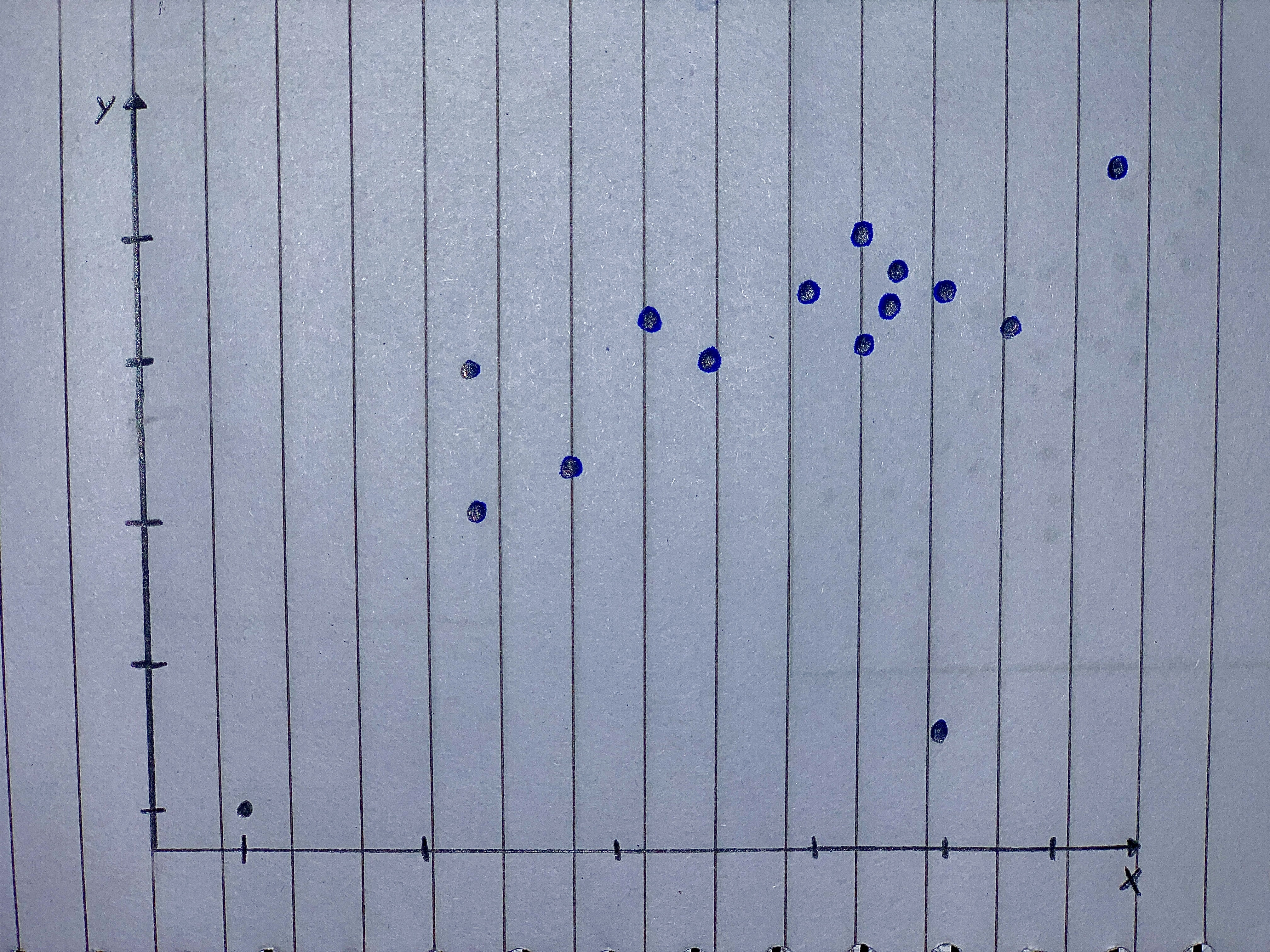Unusual points Each of the four scatterplots that follow shows a cluster of points and one “stray” point. For each, answer these questions:
1) In what way is the point unusual? Does it have high leverage, a large residual, or both?
2) Do you think that point is an influential point?
3) If that point were removed, would the correlation be- come stronger or weaker? Explain.
4) If that point were removed, would the slope of the re- gression line increase or decrease? Explain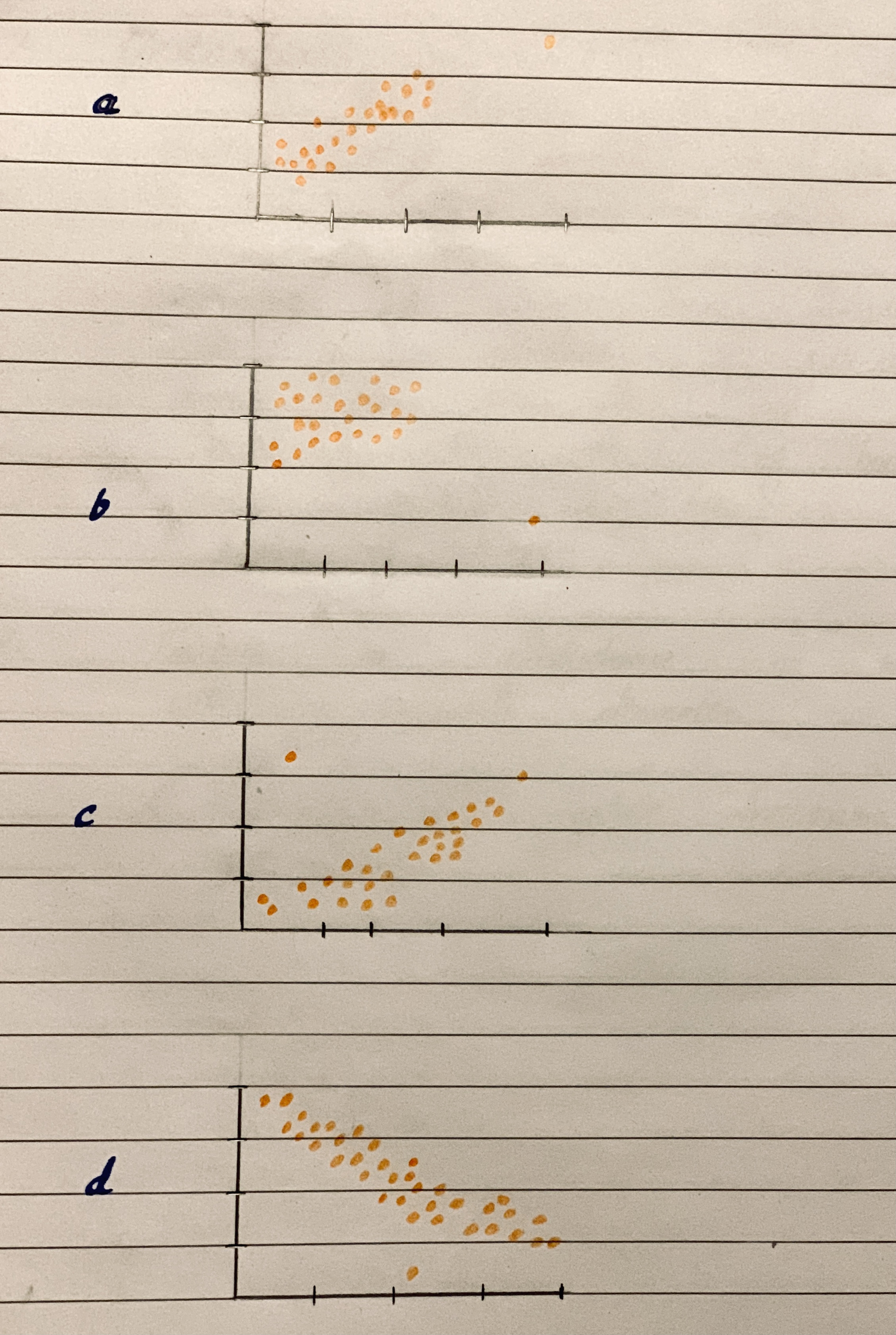Matching Here are several scatterplots. The calculated correlations are -0.923, -0.487, 0.006, and 0.777.
Which is which?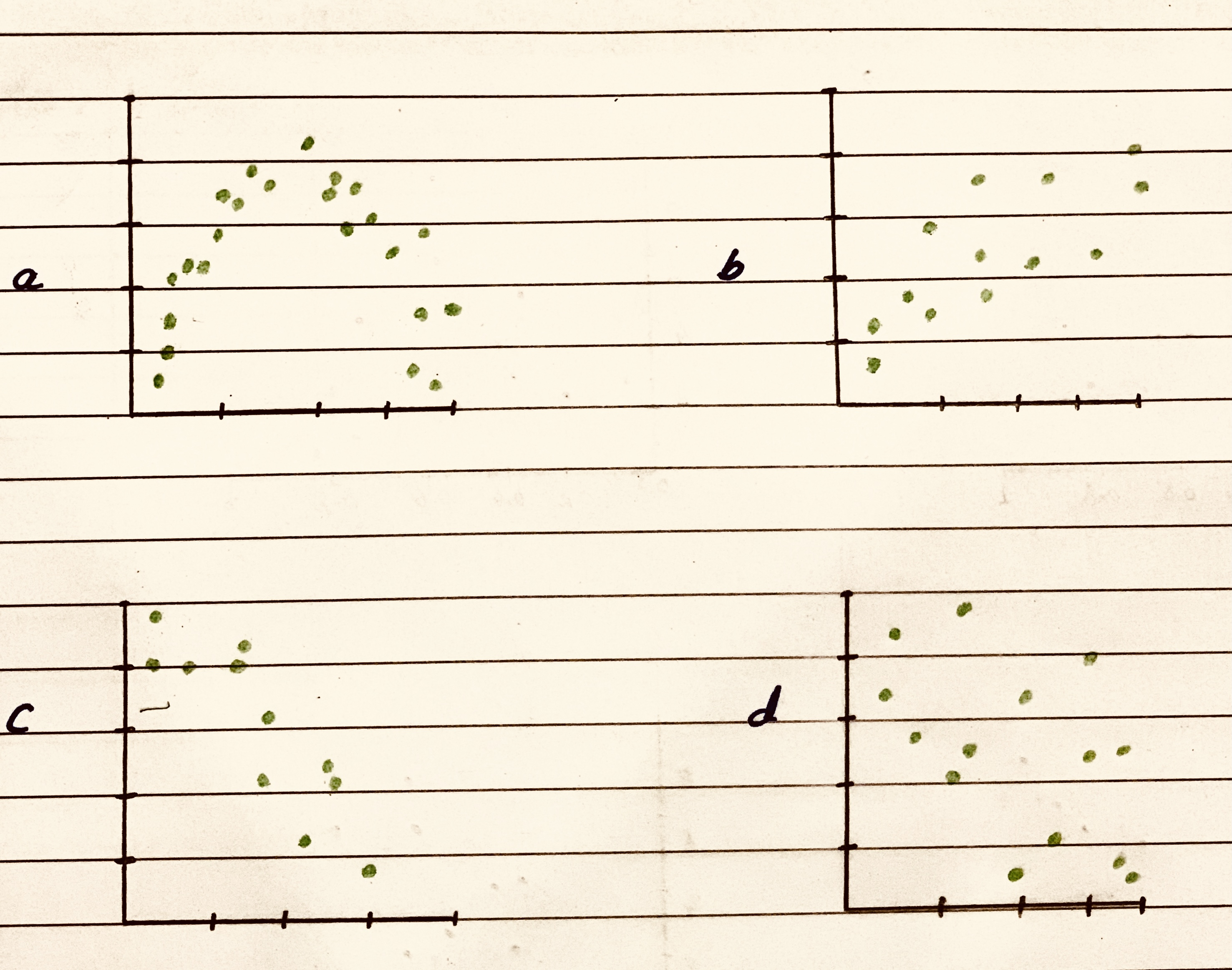Scatterplots Which of the scatterplots below show
a) little or no association?
b) a negative association?
c) a linear association?
d) a moderately strong association?
e) a very strong association?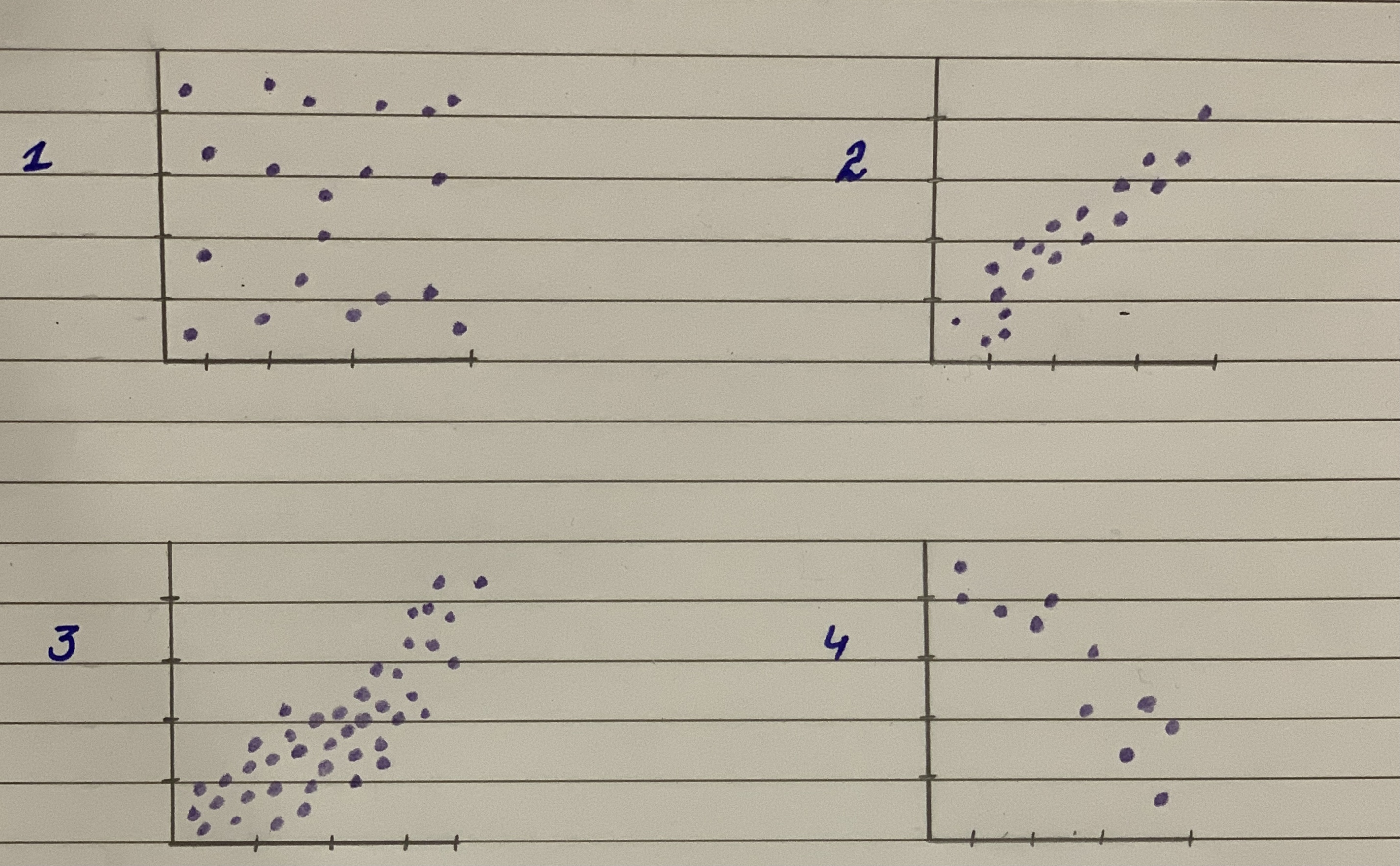Predicting Land Value Both figures concern the assessed value of land (with homes on the land), and both use the same data set. (a). Which do you think has a stronger relationship with value of the land-the number of acres of land or the number of rooms n the homes? Why? b. Il you were trying to predict the value of a parcel of land in e arca (on which there is a home), would you be able to te a better prediction by knowing the acreage or the num- ber of rooms in the house? Explain. (Source: Minitab File, Student 12. "Assess.")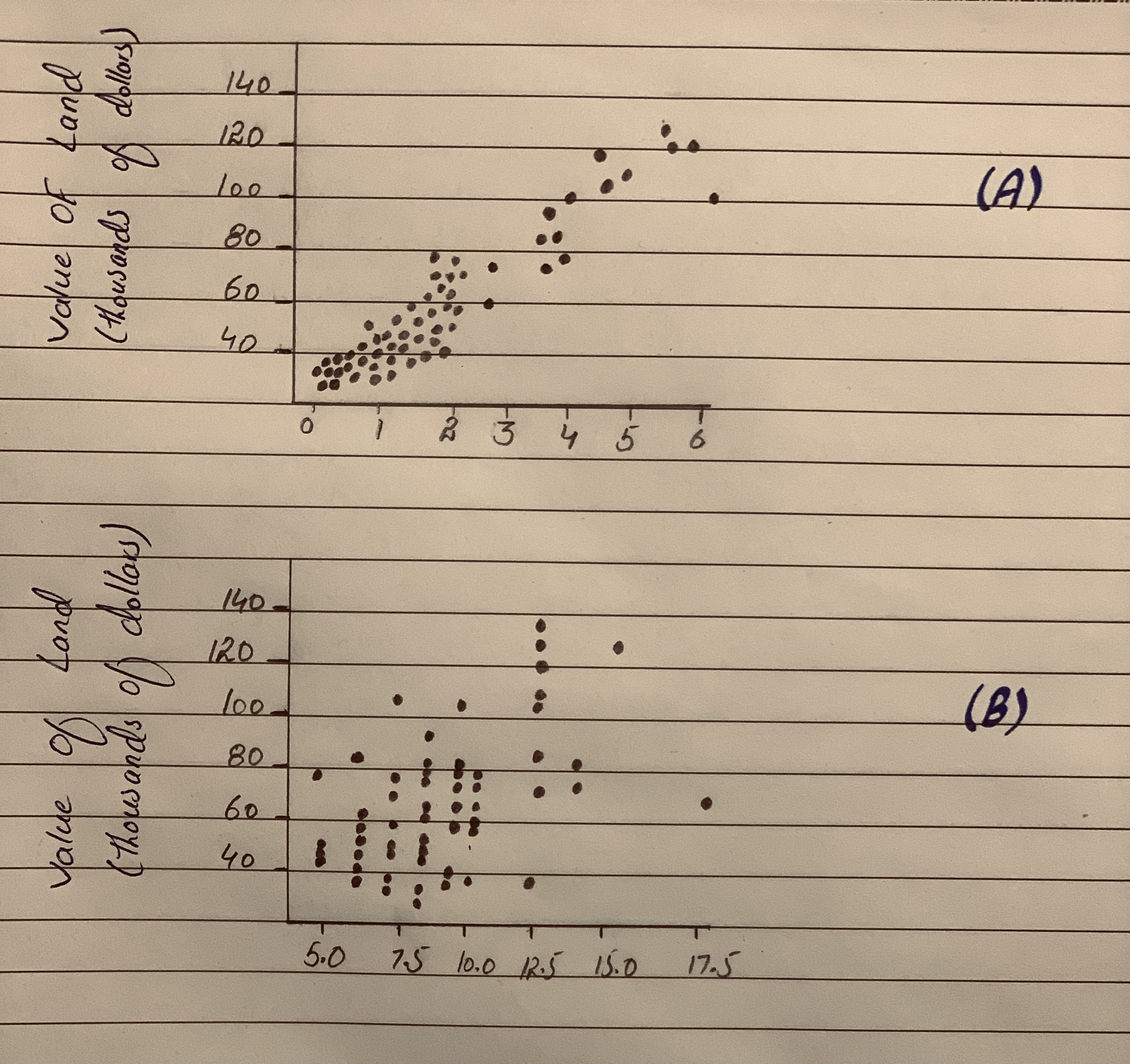Horace keeps track of the amount of time he studies and the score he gets on his quizzes. The data are shown in the table below. Which of the scatter plots below accurately records the data?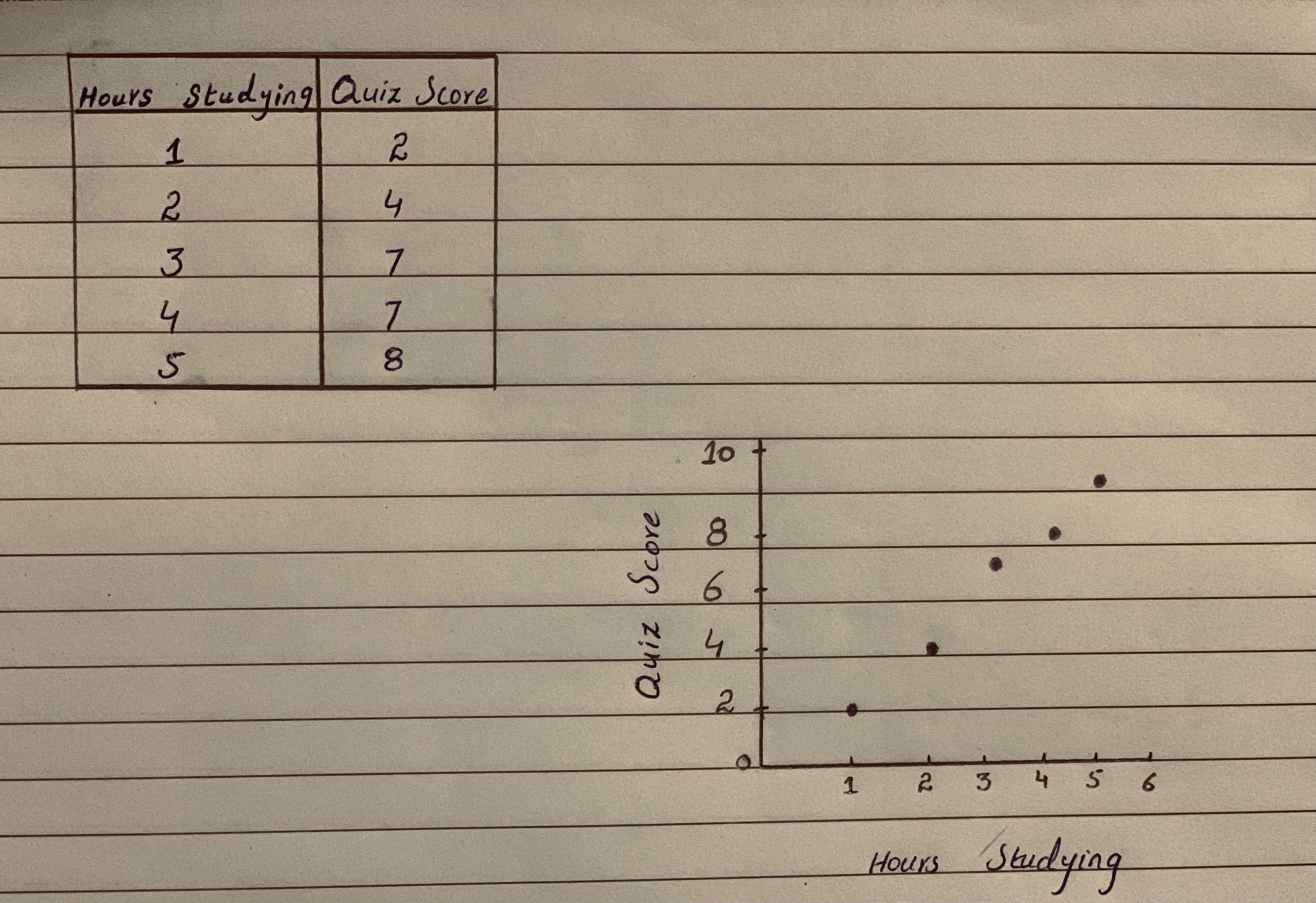Which two of the four scatter plots have most positive correlation and closer to zero correlation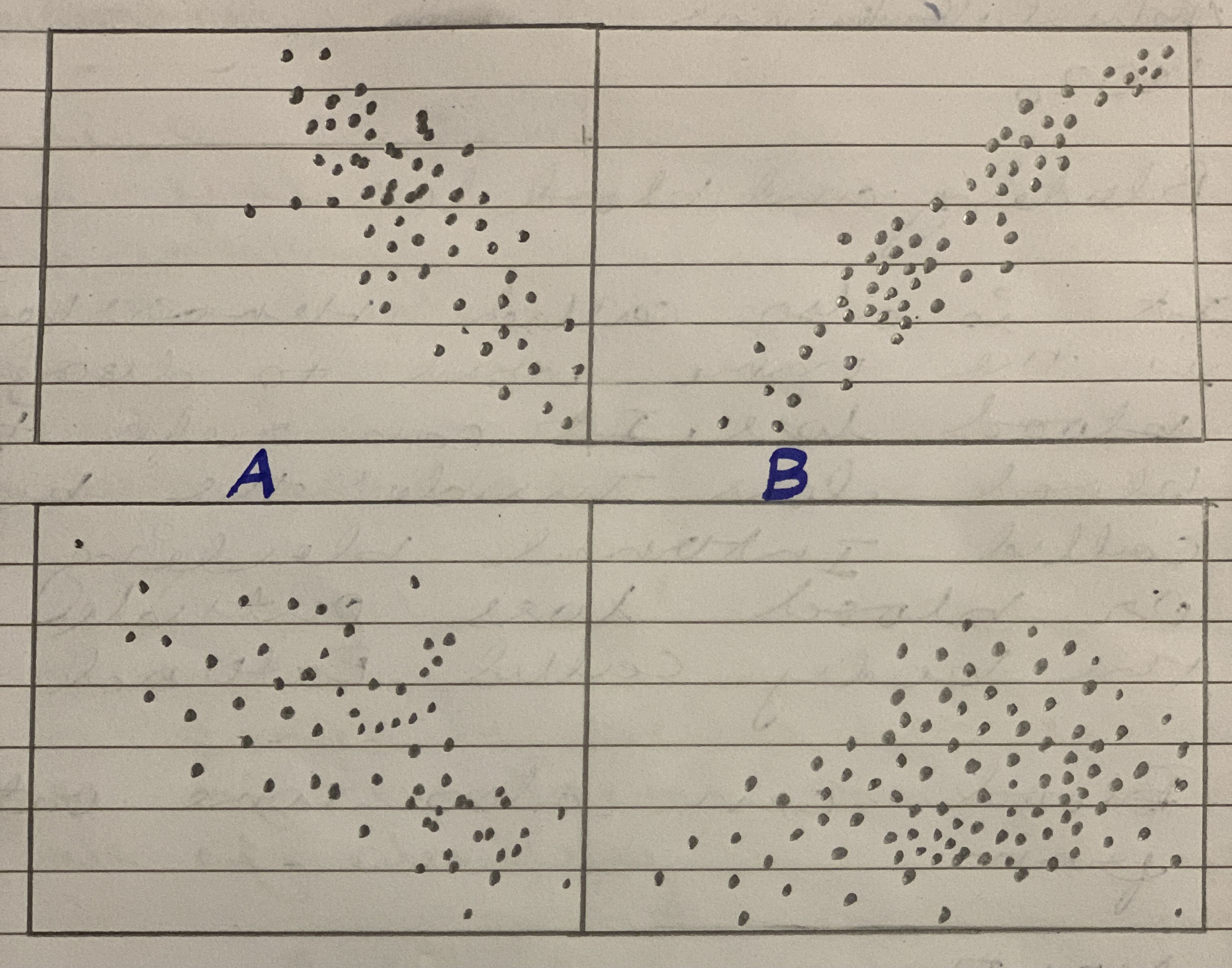We have to explain the relationship between employee performance 01 and employee performance 02 using the scater plot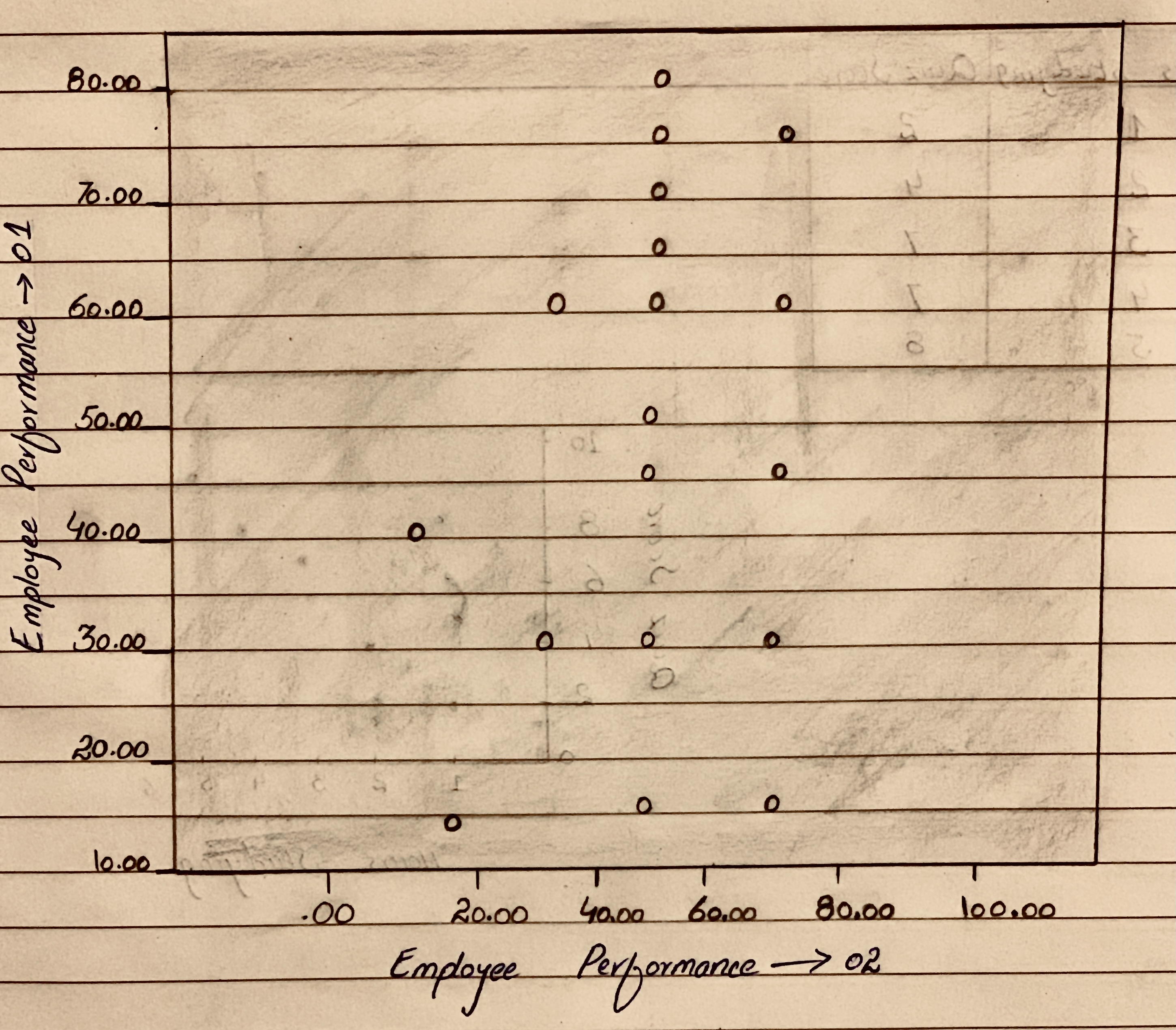The accompanying data on y = normalized energy $$\displaystyle{\left[{\left(\frac{{J}}{{m}^{{2}}}\right)}\right]}$$ and x = intraocular pressure (mmHg) appeared in a scatterplot in the article “Evaluating the Risk of Eye Injuries: Intraocular Pressure During High Speed Projectile Impacts” (Current Eye Research, 2012: 43–49), an estimated regression function was superimposed on the plot.
x 2761 19764 25713 3980 12782 19008 y 1553 14999 32813 1667 8741 16526 x 19028 14397 9606 3905 25731 y 26770 16526 9868 6640 1220 30730
Here is Minitab output from fitting the simple linear regression model. Does the model appear to specify a useful relationship between the two variables?
Predictor Coef SE Coef T P Constant -5090 2257 -2.26 0.048 Pressure 1.2912 0.1347 9.59 0.000Two scatterplots are shown below.
Scatterplot 1
A scatterplot has 14 points.
The horizontal axis is labeled "x" and has values from 30 to 110.
The vertical axis is labeled "y" and has values from 30 to 110.
The points are plotted from approximately (55, 60) up and right to approximately (95, 85).
The points are somewhat scattered.
Scatterplot 2
A scatterplot has 10 points.
The horizontal axis is labeled "x" and has values from 30 to 110.
The vertical axis is labeled "y" and has values from 30 to 110.
The points are plotted from approximately (55, 55) steeply up and right to approximately (70, 90), and then steeply down and right to approximately (85, 60).
The points are somewhat scattered.
Explain why it makes sense to use the least-squares line to summarize the relationship between x and y for one of these data sets but not the other.
Scatterplot 1 seems to show a relationship between x and y, while Scatterplot 2 shows a relationship between the two variables. So it makes sense to use the least squares line to summarize the relationship between x and y for the data set in , but not for the data set in .
...